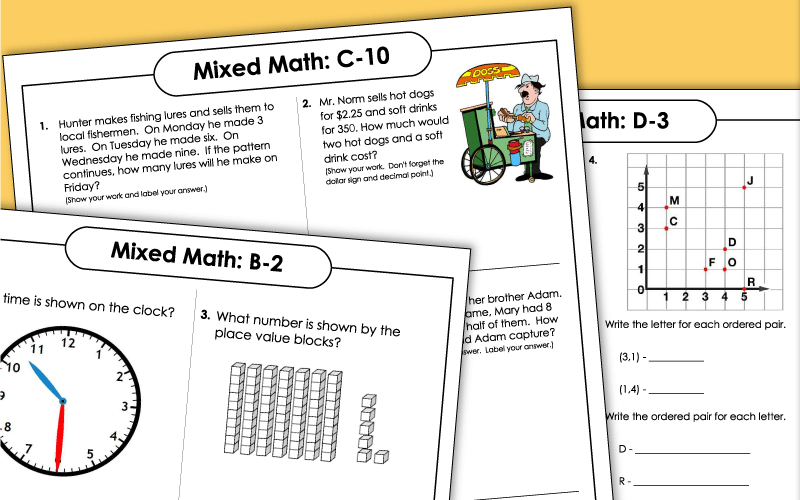# Math Word Problems (Mixed Skills)

Review mixed math skills each day with these word problem worksheets. Worksheets are sorted by grade level and require students to practice a variety of different math skills on each sheet.## Mixed Math(Level B: Grade 2)

Tell how much money is shown. Write the numbers in standard form. Solve the addition and subtraction word problems.
What number is shown by the place value blocks? Subtract to find out how many boys are in Miss Minn's class. Write the time shown on the clock.
Give values of the digits in three-digit number. Subtract to find out how many tulips are in a garden. Color the odd and even tiled numbers.
Write how much money is left after a purchase. Write the time from a clock. Choose geometric shapes.
Count by 3's. Preform skip counting. Addition and subtraction.
Fractions in a circle. Count coins and subtract money word problems.
Count how many marbles Peyton has after his brother and sister give him more. How much money is left after a buying a movie ticket.
Find dates on a calendar. Complete addition and subtraction skills with a shape.
Measurement with ruler along with adding and subtracting. What fraction of the marbles are black?
Find the missing numbers. Geometry and time challenges.

## Mixed Math(Level C: Grade 3)

This mixed review worksheet includes addition and subtraction problems, as well as a coin-counting activity.
This math page contains problems relating to addition, subtraction, and telling time.
To solve these problems, students must use subtraction skills and their knowledge of place value.
Practice subtracting numbers, counting money, and writing large numbers in standard form.
This worksheet features problems that require column addition, understanding of elapsed time, and knowledge of odd/even numbers.
Review adding and subtracting, dozens, and elapsed time.
These review word problems require student knowledge of place value and rounding.
Comparing numbers using symbols. Fill in remaining number patterns. Fractions with pizza word problem.
Number patterns, solve word problem with subtraction. Fractions using tiles.

## Mixed Math(Level D: Grade 4)

Math skills for adding, subtracting and multiplying. Complete word problems with fractions. Write place values from word problems.
Complete number patterns from examples. Solve money with subtraction. Provide time shown on the clock and in 20 minutes.
Find the perimeter of the polygon. Subtraction with thousands. Ordered pairs with a graph.
Word Problems: Multi-Step

Word problems where students use reasoning and critical thinking skill to solve each problem.

Math Word Problems (by Type)

These word problems are sorted by type: addition, subtraction, multiplication, division, fractions and more.

Math Worksheets - Full Index

We have thousands of math worksheets on STW, on nearly every topic imaginable. Check out our complete math index.

## Sample Worksheet ImagesMy Account
Site Information